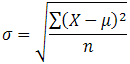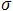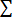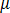# Measures of Dispersion

Measures of dispersion provide information about how much variation there is in the data, including the range, inter-quartile range and the standard deviation.

### Range

The range is simply the difference between the maximum and minimum values in a data set.

Range = max - min

So in a data set of 2, 2, 3, 4, 5, 5, 6, 7, 8, 9, 11, 13, 15, 15, 17, 19, 20, the range is the difference between 2 and 20.

18 = 20 - 2

While it is useful in seeing how large the difference in observations is in a sample, it says nothing about the spread of the data.

### Inter-quartile range

The inter-quartile range (IQR) gives more information about how the observation values of a data set are dispersed. It shows the range of the middle 50% of observations.

The IQR is found by first finding the median (see Measures of Central Tendency) of a data set, then by finding the medians of the bottom 50% of the data and the top 50% of the data.

1. Find the median: 2, 2, 3, 4, 5, 5, 6, 7, 8, 9, 11, 13, 15, 15, 17, 19, 20
2. Find the first quartile (Q1), which is the median of the bottom 50%: 2, 2, 3, 4, 5, 5, 6, 7, 8
3. Find the second quartile (Q2), which is the median of the top 50%: 8, 9, 11, 13, 15, 15, 17, 19, 20

The IQR is the difference between Q1 and Q2.

IQR = Q2 - Q1

​10 = 15 - 5

The IQR is a necessary measure of spread when using the median as a measure of central tendency.

### Standard Deviation

The standard deviation indicates the average distance between an observation value, and the mean of a data set. In this way, it shows how well the mean represents the values in a data set. Like the mean, it is appropriate to use when the data set is not skewed or containing outliers.

The formula for calculating the standard deviation of a sample is:= population standard deviation= sum of...= population mean
n = number of scores in sample.

(taken from Laerd Statistics, 2013)

However the standard deviation can be easily calculated with statistical programs and online calculators.

### Source

Laerd Statistics (2013). 'Standard deviation' [Webpage]. Retrieved from https://statistics.laerd.com/statistical-guides/measures-of-spread-standard-deviation.php

This page is a stub (a minimal version of a page). You can help expand it. Click on or to suggest additional resources, share your experience using the option, or volunteer to expand the description.

Updated: 15th August 2014 - 3:58pm
This Option is useful for:
A special thanks to this page's contributors

## Comments

There are currently no comments. Be the first to comment on this page!

## Add new comment

Login Login and comment as BetterEvaluation member or simply fill out the fields below.